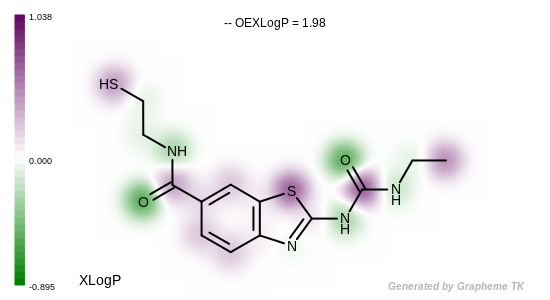# OEGetXLogPResult¶

```OEGetXLogPResult(OEMolBase mol, OEFloatArray atomxlogps=None) -> OEXLogPResult
```

Returns an `OEXLogPResult` object. The object contains the XLogP for the given molecule as described in the LogP section and a boolean flag indicating whether or not the computation is valid. The main reason the computation may fail is if one or more atoms are not parameterized. The returned value will be equal to the sum on the individual atom contributions plus the linear regression constant `-0.127`.

Hint

XLogP should be calculated on the neutral form of the molecule. Therefore, calling the `OERemoveFormalCharge` function of Quacpac TK is highly recommended prior to the XLogP calculation.

The `atomxlogps` parameter can be used to retrieve the contribution of each atom to the total XLogP as shown in `Listing 2`. See example in Figure: Example of depicting the atom contributions of the XLogP.

This function exists to make the calling by wrapped languages more convenient. This function should be preferred over the original `OEGetXLogP` function for wrapped languages.

Listing 2: Example of retrieving individual atom contributions to XLogP

```atomXLogP = oechem.OEFloatArray(mol.GetMaxAtomIdx())
result = oemolprop.OEGetXLogPResult(mol, atomXLogP)

if (result.IsValid()):
print("XLogP =", result.GetValue())
for atom in mol.GetAtoms():
idx = atom.GetIdx()
print(idx, atomXLogP[idx])
else:
print("XLogP failed for molecule")
```Example of depicting the atom contributions of the XLogP

`OEXLogPResult` class LogP section